# Galois field

(diff) ← Older revision | Latest revision (diff) | Newer revision → (diff)

finite field

A field with a finite number of elements. First considered by E. Galois .

The number of elements of any Galois field is a power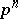of a prime number, which is the characteristic of this field. For any prime numberand any natural numberthere exists a (unique up to an isomorphism) field ofelements. It is denoted by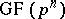or by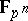. The field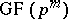contains the fieldas a subfield if and only ifis divisible by. In particular, any fieldcontains the field, which is called the prime field of characteristic. The field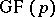is isomorphic to the fieldof residue classes of the ring of integers modulo. In any fixed algebraic closureofthere exists exactly one subfieldfor each. The correspondenceis an isomorphism between the lattice of natural numbers with respect to division and the lattice of finite algebraic extensions (in) ofwith respect to inclusion. The lattice of finite algebraic extensions of any Galois field within its fixed algebraic closure is such a lattice.

The algebraic extensionis simple, i.e. there exists a primitive elementsuch that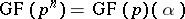. Such anwill be any root of any irreducible polynomial of degreefrom the ring. The number of primitive elements of the extensionequalswhereis the Möbius function. The additive group of the fieldis naturally endowed with the structure of an-dimensional vector space over. As a basis one may take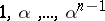. The non-zero elements ofform a multiplicative group,, of order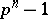, i.e. each element ofis a root of the polynomial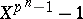. The group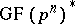is cyclic, and its generators are the primitive roots of unity of degree, the number of which is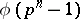, whereis the Euler function. Each primitive root of unity of degreeis a primitive element of the extension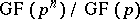, but the converse is not true. More exactly, out of theirreducible unitary polynomials of degreeoverthere are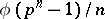polynomials of which the roots are generators of.

The set of elements ofcoincides with the set of roots of the polynomialin, i.e.is characterized as the subfield of elements fromthat are invariant with respect to the automorphism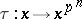, which is known as the Frobenius automorphism. If, the extension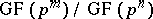is normal (cf. Extension of a field), and its Galois groupis cyclic of order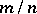. The automorphismmay be taken as the generator of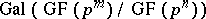.

How to Cite This Entry:
Galois field. Encyclopedia of Mathematics. URL: http://encyclopediaofmath.org/index.php?title=Galois_field&oldid=12669
This article was adapted from an original article by A.I. Skopin (originator), which appeared in Encyclopedia of Mathematics - ISBN 1402006098. See original article